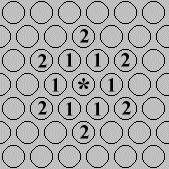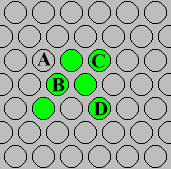## The Rules of Propagation

#####The rules that govern the behavior of each cell or space in HexLife take into account the cell/space's 12 nearest neighbors. In the illustration above, the nearest neighbors of the space marked by "*"  are the numbered spaces. There are six '1st tier neighbors', denoted by "1", and six '2nd tier neighbors', denoted by "2". As in Conway's Life, whether a cell is born, dies, or survives to the next generation is determined by how many of these 12 neighboring spaces contain live cells. Each generation the number of live neighbors of each space are added up. However, live 2nd tier neighbors are only weighted as 0.3 in this sum whereas live 1st tier neighbors are weighted as 1.0. A cell is born into an empty space if this sum falls within the range of 2.3 - 2.9. Otherwise the space remains empty. A live cell survives to the next generation if this sum falls within the range of 2.0 - 3.3. Otherwise it dies (becomes an empty space).This illustration shows a simple pattern of live cells (green circles). The sum of live neighbors for space "A" is 2.6 (2 1st tier neighbors x 1.0 plus 2 2nd tier neighbors x 0.3 = 2.0 + 0.6 = 2.6). In the next generation there will a new cell in this space. The sum for cell "B" is 3.6. This cell will die from overcrowding. Cell "C" will survive to the next generation as its sum is 2.6. Cell "D" will die, on the other hand, since its sum is only 1.6.